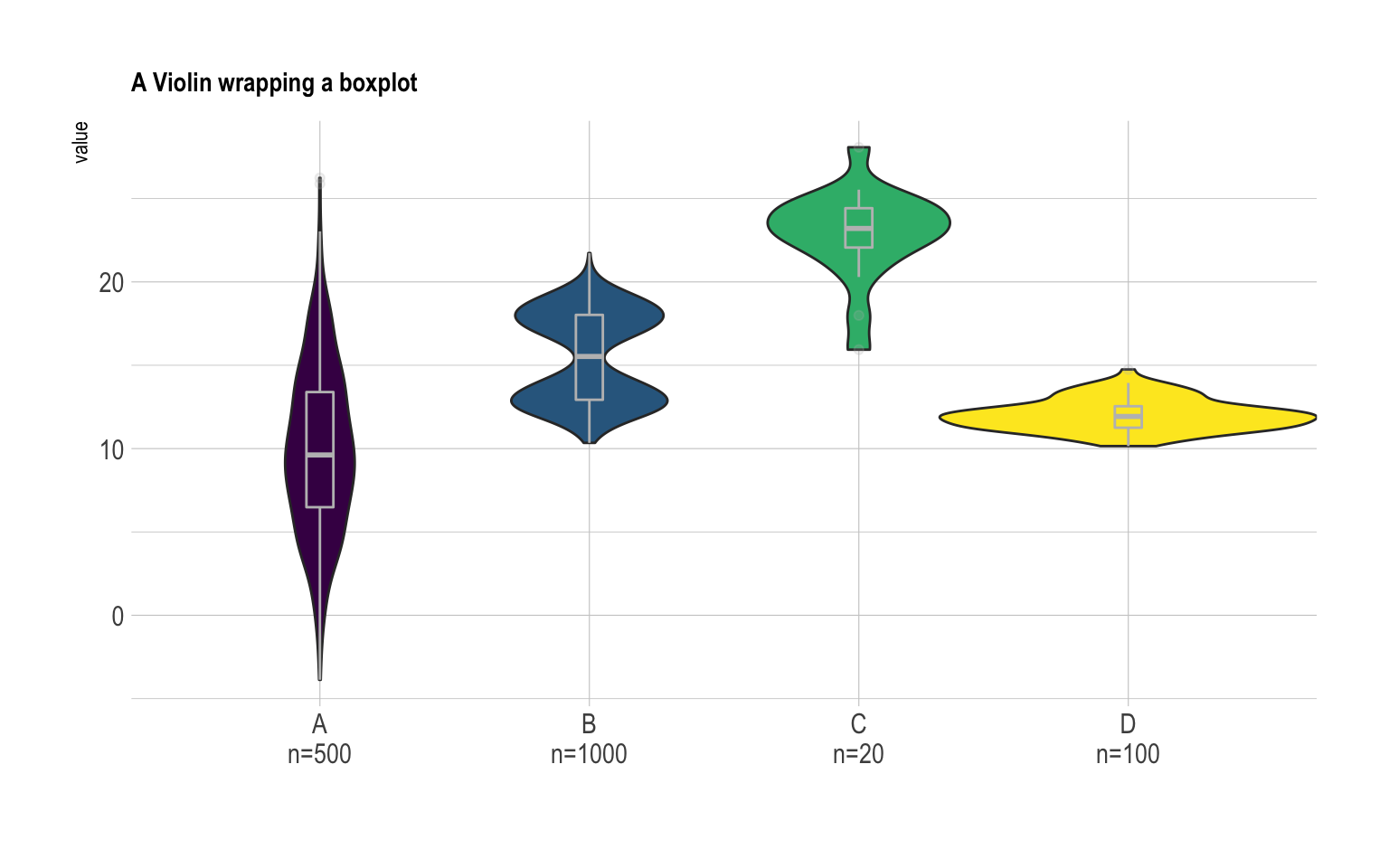# Violin plot with included boxplot and sample size in ggplot2

It can be handy to include a boxplot in the violin plot to see both the distribution of the data and its summary statistics. Moreover, adding sample size of each group on the X axis is often a necessary step. Here is how to do it with `R` and ggplot2.

Building a violin plot with `ggplot2` is pretty straightforward thanks to the dedicated `geom_violin()` function. It is possible to use `geom_boxplot()` with a small `width` in addition to display a boxplot that provides summary statistics.

Moreover, note a small trick that allows to provide sample size of each group on the X axis: a new column called `myaxis` is created and is then used for the X axis.

``````# Libraries
library(ggplot2)
library(dplyr)
library(hrbrthemes)
library(viridis)

# create a dataset
data <- data.frame(
name=c( rep("A",500), rep("B",500), rep("B",500), rep("C",20), rep('D', 100)  ),
value=c( rnorm(500, 10, 5), rnorm(500, 13, 1), rnorm(500, 18, 1), rnorm(20, 25, 4), rnorm(100, 12, 1) )
)

# sample size
sample_size = data %>% group_by(name) %>% summarize(num=n())

# Plot
data %>%
left_join(sample_size) %>%
mutate(myaxis = paste0(name, "\n", "n=", num)) %>%
ggplot( aes(x=myaxis, y=value, fill=name)) +
geom_violin(width=1.4) +
geom_boxplot(width=0.1, color="grey", alpha=0.2) +
scale_fill_viridis(discrete = TRUE) +
theme_ipsum() +
theme(
legend.position="none",
plot.title = element_text(size=11)
) +
ggtitle("A Violin wrapping a boxplot") +
xlab("")``````Related chart types

## Contact

This document is a work by Yan Holtz. Any feedback is highly encouraged. You can fill an issue on Github, drop me a message on Twitter, or send an email pasting yan.holtz.data with gmail.com.